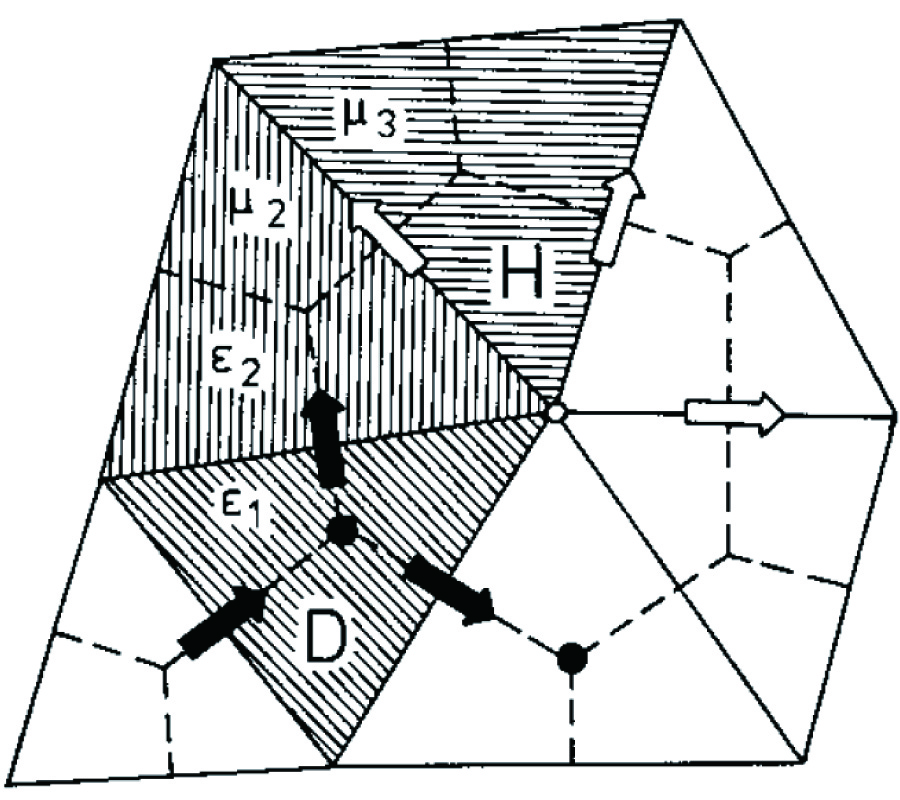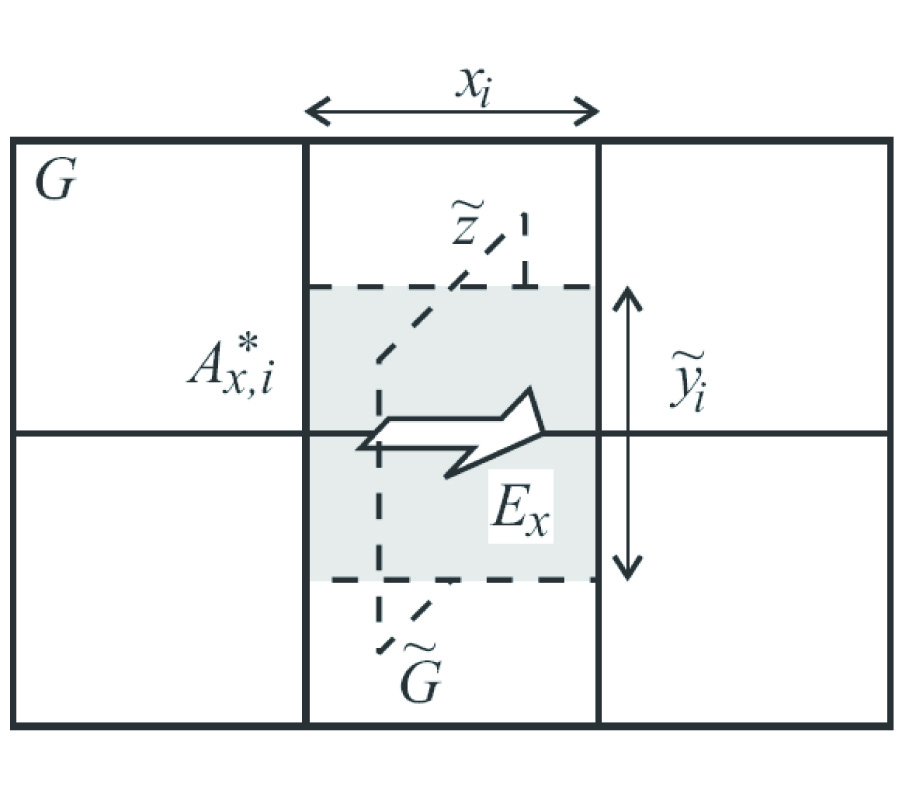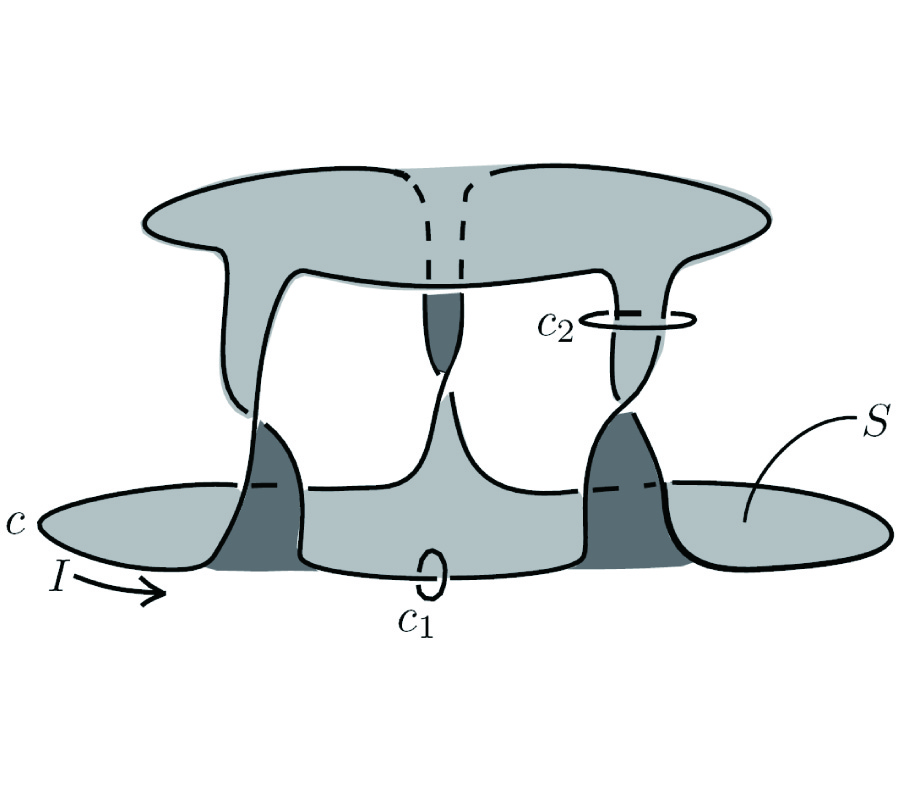ISSN: 1070-4698

# Vol. 32

Latest Volume
All Volumes
All Issues

PIER Vol. 32, 413-428, 2001. doi:10.2528/PIER00080116

### Covariant Isotropic Constitutive Relations in Clifford's Geometric Algebra

Henri Puska

Constitutive relations for isotropic material media are formulated in a manifestly covariant manner. Clifford's geometric algebra is used throughout. Polarisable,c hiral and Tellegen medium are investigated. The investigation leads to the discovery of an underlying algebraic structure that completely classifies isotropic media. Variational properties are reviewed,sp ecial attention is paid to the imposed constraints on material parameters. Covariant reciprocity condition is given. Finally,dualit y transformations and their relevance to constitutive relations are investigated. Duality is shown to characterise ‘well-behavedness' of medium which has an interesting metric tensor related implication.

PIER Vol. 32, 383-411, 2001. doi:10.2528/PIER00080115

### Implementing the Perfectly Matched Layer Absorbing Boundary Condition with Mimetic Differencing Schemes

M. W. Buksas

This paper concerns the implementation of the perfectly matched layer (PML) absorbing boundary condition in the framework of a mimetic differencing scheme for Maxwell's Equations. We use mimetic versions of the discrete curl operator on irregular logically rectangular grids to implement anisotropic tensor formulation of the PML. The form of the tensor we use is fixed with respect to the grid and is known to be perfectly matched in the continuous limit for orthogonal coordinate systems in which the metric is constant, i.e. Cartesian coordinates, and quasi-perfectly matched (quasi-PML) for curvilinear coordinates. Examples illustrating the effectiveness and long-term stability of the methods are presented. These examples demonstrate that the grid-based coordinate implementation of the PML is effective on Cartesian grids, but generates systematic reflections on grids which are orthogonal but non-Cartesian (quasi-PML). On non-orthogonal grids progressively worse performance of the PML is demonstrated. The paper begins with a summary derivation of the anisotropic formulation of the perfectly matched layer and mimetic differencing schemes for irregular logically rectangular grids.

PIER Vol. 32, 357-381, 2001. doi:10.2528/PIER00080114

###Frequency Domain Analysis of Waveguides and Resonators with Fit on Non-Orthogonal Triangular Grids

Ursula van Rienen

The focus of this paper is on the solution of Maxwell's equations for time-harmonic fields on triangular, possibly nonorthogonal meshes. The method is based on the well-known Finite Integration Technique (FIT) [33, 35] which is a proven consistent discretization method for the computation of electromagnetic fields. FIT on triangular grids was first introduced in [29, 31] for eigenvalue problems arising in the design of accelerator components and dielectric loaded waveguides. For many technical applications the 2D simulation on a triangular grid combines the advantages of FIT, as e.g. the consistency of the method or the numerical advantage of banded system matrices, with the geometrical flexibility of non-coordinate grids. The FIT-discretization on non-orthogonal 2D grids has close relations  to the N´ed´elec elements [14, 15] or edge elements in the Finite Element Method.

PIER Vol. 32, 317-356, 2001. doi:10.2528/PIER00080113

###Computational Aspects of the Cell Method in Electrodynamics

M. Marrone

A desire to unify the mathematical description of many physical theories, such as electrodynamics, mechanics, thermal conduction, has led us to understand that global (=integral) physical variables of eachth eory can be associated to spatial geometrical elements suchas points, lines, surfaces, volumes and temporal geometrical elements suchas instants and intervals. This association has led us to build a space-time classification diagram of variables and equations for each theory. Moreover, the possibility to express physical laws directly in a finite rather than differential form has led to the development of a computational methodology called Cell Method . This paper discusses some practical aspects of this method and how it may overcome some of the main limitations of FDTD method in electrodynamics. Moreover, we will provide some numerical examples to compare the two methodologies.

PIER Vol. 32, 301-316, 2001. doi:10.2528/PIER00080112

###Conservation of Discrete Energy and Related Laws in the Finite Integration Technique

R. Schuhmann and Thomas Weiland

We report some properties of the Finite Integration Technique (FIT), which are related to the definition of a discrete energy quantity. Starting with the well-known identities for the operator matrices of the FIT, not only the conservation of discrete energy in time and frequency domain simulations is derived, but also some important orthogonality properties for eigenmodes in cavities and waveguides. Algebraic proofs are presented, which follow the vectoranalytical proofs of the related theorems of the classical (continuous) theory. Thus, the discretization approach of the FIT can be considered as the framework for a consistent discrete electromagnetic field theory.

PIER Vol. 32, 271-299, 2001. doi:10.2528/PIER00080111

### Higher Order Whitney Forms

R. Hiptmair

The calculus of differential forms can be used to devise a unified description of discrete differential forms of any order and polynomial degree on simplicial meshes in any spatial dimension. A general formula for suitable degrees of freedom is also available. Fundamental properties of nodal interpolation can be established easily. It turns out that higher order spaces, including variants with locally varying polynomial order, emerge from the usual Whitneyforms by local augmentation. This paves the way for an adaptive pversion approach to discrete differential forms.

PIER Vol. 32, 247-269, 2001. doi:10.2528/PIER00080110

###Discrete Hodge-Operators: an Algebraic Perspective

R. Hiptmair

Discrete differential forms should be used to deal with the discretization of boundary value problems that can be stated in the calculus of differential forms. This approach preserves the topological features of the equations. Yet, the discrete counterparts of the metricdependent constitutive laws remain elusive. I introduce a few purely algebraic constraints that matrices associated with discrete material laws have to satisfy. It turns out that most finite element and finite volume schemes comply with these requirements. Thus convergence analysis can be conducted in a unified setting. This discloses basic sufficient conditions that discrete material laws have to meet in order to ensure convergence in the relevant energy norms.

PIER Vol. 32, 207-245, 2001. doi:10.2528/PIER00080109

###Finite Element-Based Algorithms to Make Cuts for Magnetic Scalar Potentials: Topological Constraints and Computational Complexity

Paul W. Gross and Peter Robert Kotiuga

This paper outlines a generic algorithm to generate cuts for magnetic scalar potentials in 3-dimensional multiply-connected finite element meshes. The algorithm is based on the algebraic structures of (co)homology theory with differential forms and developed in the context of the finite element method and finite element data structures. The paper also studies the computational complexity of the algorithm and examines how the topology of the region can create an obstruction to finding cuts in O(m20) time and O(m0) storage, where m0 is the number of vertices in the finite element mesh. We argue that in a problem where there is no a priori data about the topology, the algorithm complexity is O(m20) in time and O(m4/30 ) in storage. We indicate how this complexity can be achieved in implementation and optimized in the context of adaptive mesh refinement.

PIER Vol. 32, 189-206, 2001. doi:10.2528/PIER00080108

###Topological Approach to Computational Electromagnetism

Timo Tarhasaari and Lauri Kettunen

Software systems designed to solve Maxwell's equations need abstractions that accurately explain what different kinds of electromagnetic problems really do have in common. Computational electromagnetics calls for higher level abstractions than what is typically needed in ordinary engineering problems. In this paper Maxwell's equations are described by exploiting basic concepts of set theory. Although our approach unavoidably increases the level of abstraction,it also simplifies the overall view making it easier to recognize a topological problem behind all boundary value problems modeling the electromagnetic phenomena. This enables us also to construct an algorithm which tackles the topological problem with basic tools of linear algebra.

PIER Vol. 32, 171-188, 2001. doi:10.2528/PIER00080107

### Geometric Aspects of the Simplicial Discretization of Maxwell's Equations

Fernando Lisboa Teixeira

Aspects of the geometric discretization of electromagnetic fields on simplicial lattices are considered. First, the convenience of the use of exterior differential forms to represent the field quantities through their natural role as duals (cochains) of the geometric constituents of the lattice (chains = nodes, edges, faces, volumes) is briefly reviewed. Then, the use of the barycentric subdivision to decompose the (ordinary) simplicial primal lattice together with the (twisted) non-simplicial barycentric dual lattice into simplicial elements is considered. Finally, the construction of lattice Hodge operators by using Whitney maps on the first barycentric subdivision is described. The objective is to arrive at a discrete formulation of electromagnetic fields on general lattices which better adheres to the underlying physics.

PIER Vol. 32, 151-169, 2001. doi:10.2528/PIER00080106

###Data Structures for Geometric and Topological Aspects of Finite Element Algorithms

Paul W. Gross and Peter Robert Kotiuga

This paper uses simplicial complexes and simplicial (co)homology theory to expose a foundation for data structures for tetrahedral finite element meshes. Identifying tetrahedral meshes with simplicial complexes leads, by means of Whitney forms, to the connection between simplicial cochains and fields in the region modeled by the mesh. Furthermore, lumped field parameters are tied to matrices associated with simplicial (co)homology groups. The data structures described here are sparse, and the computational complexity of constructing them is O(n) where n is the number of vertices in the finite element mesh. Non-tetrahedral meshes can be handled by an equivalent theory. These considerations lead to a discrete form of Poincar´e duality which is a powerful tool for developing algorithms for topological computations on finite element meshes. This duality emerges naturally in the data structures. We indicate some practical applications of both data structures and underlying theory.

PIER Vol. 32, 123-149, 2001. doi:10.2528/PIER00080105

###The Geometry of Time-Stepping

C. Mattiussi

The space-time geometric structure of Maxwell's equations is examined and a subset of them is found to define a pair of exact discrete time-stepping relations. The desirability of adopting an approach to the discretization of electromagnetic problems which exploits this fact is advocated, and the name topological time-stepping for numerical schemes complying with it is suggested. The analysis of the equations leading to this kind of time-stepping reveals that these equations are naturally written in terms of integrated field quantities associated with space-time domains. It is therefore suggested that these quantities be adopted as state variables within numerical methods. A list of supplementary prescriptions for a discretization of electromagnetic problems suiting this philosophy is given, with particular emphasis on the necessity to adopt a space-time approach in each discretization step. It is shown that some existing methods already comply with these tenets, but that this fact is not explicitly recognized and exploited. The role of the constitutive equations in this discretization philosophy is briefly analyzed. The extension of this approach to more general kinds of space-time meshes, to other sets of basic time-stepping equations and to other field theories is finally considered.

PIER Vol. 32, 89-121, 2001. doi:10.2528/PIER00080104

###Mimetic Finite Difference Methods for Maxwell's Equations and the Equations of Magnetic Diffusion

J. M. Hyman and M. Shashkov

We have constructed mimetic finite difference methods for both the TE and TM modes for 2-D Maxwell's curl equations and equations of magnetic diffusion with discontinuous coefficients on nonorthogonal, nonsmooth grids. The discrete operators were derived using the discrete vector and tensor analysis to satisfy discrete analogs of the main theorems of vector analysis. Because the finite difference methods satisfy these theorems, they do not have spurious solutions and the "divergence-free" conditions for Maxwell's equations are automatically satisfied. The tangential components of the electric field and the normal components of magnetic flux used in the FDM are continuous even across discontinuities. This choice guarantees that problems with strongly discontinuous coefficients are treated properly. Furthermore on rectangular grids the method reduces to the analytically correct averaging for discontinuous coefficients. We verify that the convergence rate was between first and second order on the arbitrary quadrilateral grids and demonstrate robustness of the method in numerical examples.

PIER Vol. 32, 65-87, 2001. doi:10.2528/PIER00080103

###Discrete Electromagnetism with the Finite Integration Technique

Markus Clemens and Thomas Weiland

The Finite Integration Technique (FIT) is a consistent discretization scheme for Maxwell's equations in their integral form. The resulting matrix equations of the discretized fields can be used for efficient numerical simulations on modern computers. In addition, the basic algebraic properties of this discrete electromagnetic field theory allow to analytically and algebraically prove conservation properties with respect to energy and charge of the discrete formulation and gives an explanation of the stability properties of numerical formulations in the time domain.

PIER Vol. 32, 45-64, 2001. doi:10.2528/PIER00080102

### 'Generalized Finite Differences' in Computational Electromagnetics

Alain Bossavit

The geometrical approach to Maxwell's equations promotes a way to discretize them that can be dubbed "Generalized Finite Differences", which has been realized independently in several computing codes. The main features of this method are the use of two grids in duality, the "metric-free" formulation of the main equations (Amp`ere and Faraday), and the concentration of metric information in the discrete representation of the Hodge operator. The specific role that finite elements have to play in such an approach is emphasized, and a rationale for Whitney forms is proposed, showing why they are the finite elements of choice.

PIER Vol. 32, 1-44, 2001. doi:10.2528/PIER00080101

###Finite Formulation of the Electromagnetic Field

E. Tonti

The objective of this paper is to present an approach to electromagnetic field simulation based on the systematic use of the global (i.e. integral) quantities. In this approach, the equations of electromagnetism are obtained directly in a finite form starting from experimental laws without resortingto the differential formulation. This finite formulation is the natural extension of the network theory to electromagnetic field and it is suitable for computational electromagnetics.